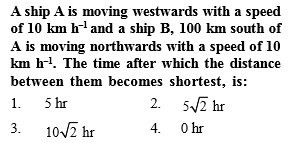Concept Videos :-

#35-Intro-to-Relative-Motion
#36-Solved-Examples-19
#37-Solved-Examples-20
#38-Concept-of-River-Man-Problem
#39-Solved-Examples-21
#40-Rain-Man-Problem
#41-Wind-Plane-Problem

Concept Questions :-

Relative motion

$\mathrm{Initially}$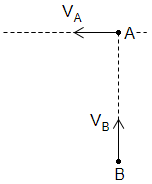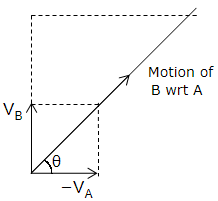$\mathrm{tan\theta }=\frac{{\mathrm{V}}_{\mathrm{B}}}{{\mathrm{V}}_{\mathrm{A}}}=1\phantom{\rule{0ex}{0ex}}\mathrm{\theta }=45°$

At shortest distance, the line dropped from A onto B should be perpendicular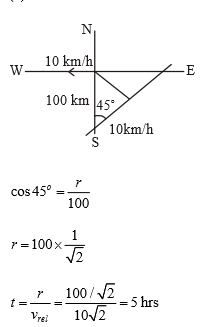Difficulty Level:

• 41%
• 29%
• 23%
• 9%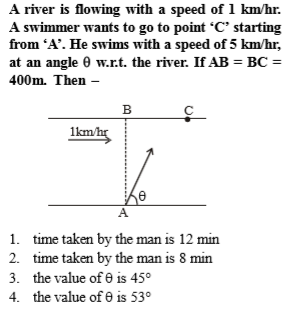Concept Videos :-

#35-Intro-to-Relative-Motion
#36-Solved-Examples-19
#37-Solved-Examples-20
#38-Concept-of-River-Man-Problem
#39-Solved-Examples-21
#40-Rain-Man-Problem
#41-Wind-Plane-Problem

Concept Questions :-

Relative motion

(4)

Difficulty Level:

• 13%
• 18%
• 39%
• 33%

A boat is sent across a river with a velocity of 8 km/hr. If the resultant velocity of boat is 10 km/hr, then velocity of the river is :

(1) 10 km/hr

(2) 8 km/hr

(3) 6 km/hr

(4) 4 km/hr

Concept Videos :-

#35-Intro-to-Relative-Motion
#36-Solved-Examples-19
#37-Solved-Examples-20
#38-Concept-of-River-Man-Problem
#39-Solved-Examples-21
#40-Rain-Man-Problem
#41-Wind-Plane-Problem

Concept Questions :-

Relative motion

(3) Given $\stackrel{\to }{AB}=$ Velocity of boat= 8 km/hr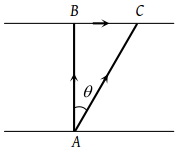$\stackrel{\to }{AC}=$ Resultant velocity of boat = 10 km/hr

$\stackrel{\to }{BC}=$Velocity of river $=\sqrt{A{C}^{2}-A{B}^{2}}$

$=\sqrt{{\left(10\right)}^{2}-{\left(8\right)}^{2}}=6\text{\hspace{0.17em}}km/hr$

Difficulty Level:

• 7%
• 11%
• 77%
• 7%

A boat moves with a speed of 5 km/h relative to water in a river flowing with a speed of 3 km/h and having a width of 1 km. The minimum time taken around a round trip is

(1) 5 min

(2) 60 min

(3) 20 min

(4) 30 min

Concept Videos :-

#35-Intro-to-Relative-Motion
#36-Solved-Examples-19
#37-Solved-Examples-20
#38-Concept-of-River-Man-Problem
#39-Solved-Examples-21
#40-Rain-Man-Problem
#41-Wind-Plane-Problem

Concept Questions :-

Relative motion

(4) For the round trip he should cross perpendicular to the river

∴ Time for trip to that side $=\frac{1km}{4km/hr}=0.25hr$

To come back, again he take 0.25 hr to cross the river.

Total time is 30 min, he goes to the other bank and come back at the same bank

Difficulty Level:

• 16%
• 18%
• 16%
• 53%

A river is flowing from W to E with a speed of 5 m/min. A man can swim in still water with a velocity 10 m/min. In which direction should the man swim so as to take the shortest possible path to go to the south.

(1) 30° with downstream

(2) 60° with downstream

(3) 120° with downstream

(4) South

Concept Videos :-

#35-Intro-to-Relative-Motion
#36-Solved-Examples-19
#37-Solved-Examples-20
#38-Concept-of-River-Man-Problem
#39-Solved-Examples-21
#40-Rain-Man-Problem
#41-Wind-Plane-Problem

Concept Questions :-

Relative motion

(3) For shortest possible path man should swim with an angle (90+θ) with downstream.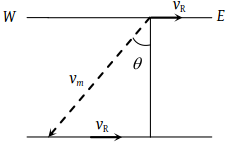From the fig, $\mathrm{sin}\theta =\frac{{v}_{r}}{{v}_{m}}=\frac{5}{10}=\frac{1}{2}$⇒ ∴ θ = 30°

So angle with downstream = $90°+30°=120°$

Difficulty Level:

• 21%
• 24%
• 49%
• 8%

A train is moving towards east and a car is along north, both with same speed. The observed direction of car to the passenger in the train is

(1) East-north direction

(2) West-north direction

(3) South-east direction

(4) None of these

Concept Videos :-

#35-Intro-to-Relative-Motion
#36-Solved-Examples-19
#37-Solved-Examples-20
#38-Concept-of-River-Man-Problem
#39-Solved-Examples-21
#40-Rain-Man-Problem
#41-Wind-Plane-Problem

Concept Questions :-

Relative motion

(2) $\stackrel{\to }{{v}_{ct}}=\stackrel{\to }{{v}_{c}}-\stackrel{\to }{{v}_{t}}$

$\stackrel{\to }{{v}_{ct}}=\stackrel{\to }{{v}_{c}}+\left(-\stackrel{\to }{{v}_{t}}\right)$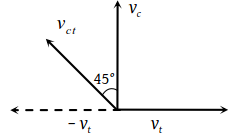Velocity of car w.r.t. train $\left({v}_{ct}\right)$ is towards West – North

Difficulty Level:

• 25%
• 55%
• 16%
• 7%

Two cars are moving in the same direction with the same speed 30 km/hr. They are separated by a distance of 5 km, the speed of a car moving in the opposite direction if it meets these two cars at an interval of 4 minutes, will be

(1) 40 km/hr

(2) 45 km/hr

(3) 30 km/hr

(4) 15 km/hr

Concept Videos :-

#35-Intro-to-Relative-Motion
#36-Solved-Examples-19
#37-Solved-Examples-20
#38-Concept-of-River-Man-Problem
#39-Solved-Examples-21
#40-Rain-Man-Problem
#41-Wind-Plane-Problem

Concept Questions :-

Relative motion

(2) The two car (say A and B) are moving with same velocity, the relative velocity of one (say B) with respect to the other $A,\text{​\hspace{0.17em}}{\stackrel{\to }{v}}_{BA}={\stackrel{\to }{v}}_{B}-{\stackrel{\to }{v}}_{A}=v-v=0$

So the relative separation between them (= 5 km) always remains the same.

Now if the velocity of car (say C) moving in opposite direction to A and B, is ${\stackrel{\to }{v}}_{C}$ relative to ground then the velocity of car C relative to A and B will be ${\stackrel{\to }{v}}_{rel.}={\stackrel{\to }{v}}_{C}-\stackrel{\to }{v}$

But as $\stackrel{\to }{v}$ is opposite to vC

So $\text{\hspace{0.17em}}{v}_{rel}={v}_{c}-\left(-30\right)=\left({v}_{C}+30\right)\text{\hspace{0.17em}}km\text{​​​}/\text{​​}hr.$

So, the time taken by it to cross the cars A and B $t=\frac{d}{{v}_{rel}}\text{​\hspace{0.17em}\hspace{0.17em}\hspace{0.17em}\hspace{0.17em}}⇒\text{\hspace{0.17em}\hspace{0.17em}\hspace{0.17em}}\frac{4}{60}=\frac{5}{{v}_{C}+30}\text{\hspace{0.17em}\hspace{0.17em}}$

$⇒\text{\hspace{0.17em}\hspace{0.17em}\hspace{0.17em}}{v}_{C}=45\text{\hspace{0.17em}}km/hr.$

Difficulty Level:

• 11%
• 74%
• 11%
• 6%

A man standing on a road hold his umbrella at 30° with the vertical to keep the rain away. He throws the umbrella and starts running at 10 km/hr. He finds that raindrops are hitting his head vertically, the speed of raindrops with respect to the road will be

(1) 10 km/hr

(2) 20 km/hr

(3) 30 km/hr

(4) 40 km/hr

Concept Videos :-

#35-Intro-to-Relative-Motion
#36-Solved-Examples-19
#37-Solved-Examples-20
#38-Concept-of-River-Man-Problem
#39-Solved-Examples-21
#40-Rain-Man-Problem
#41-Wind-Plane-Problem

Concept Questions :-

Relative motion

(2) When the man is at rest w.r.t. the ground, the rain comes to him at an angle 30° with the vertical. This is the direction of the velocity of raindrops with respect to the ground.

Here ${\stackrel{\to }{v\text{\hspace{0.17em}}}}_{rg}=$ velocity of rain with respect to the ground

${\stackrel{\to }{\text{\hspace{0.17em}}v}}_{mg}=$ velocity of the man with respect to the ground.

and ${\stackrel{\to }{\text{\hspace{0.17em}}v}}_{rm}=$ velocity of the rain with respect to the man,

We have ${\stackrel{\to }{\text{\hspace{0.17em}}v}}_{r\text{\hspace{0.17em}\hspace{0.17em}}g}={\stackrel{\to }{\text{\hspace{0.17em}}v}}_{r\text{\hspace{0.17em}}m}+{\stackrel{\to }{\text{\hspace{0.17em}}v}}_{mg}$ ......(i)

Taking horizontal components equation (i) gives

${v}_{r\text{\hspace{0.17em}​}g}\mathrm{sin}\text{\hspace{0.17em}}30°={v}_{m\text{\hspace{0.17em}}g}=10\text{\hspace{0.17em}}km/hr$

or ${v}_{r\text{\hspace{0.17em}}g}=\frac{10}{\mathrm{sin}30°}=20\text{\hspace{0.17em}}km/hr$

Difficulty Level:

• 15%
• 74%
• 10%
• 3%

A boat is moving with a velocity 3i + 4j with respect to ground. The water in the river is moving with a velocity – 3i – 4j with respect to ground. The relative velocity of the boat with respect to water is

(1) 8j

(2) – 6i – 8j

(3) 6i +8j

(4) $5\sqrt{2}$

Concept Videos :-

#35-Intro-to-Relative-Motion
#36-Solved-Examples-19
#37-Solved-Examples-20
#38-Concept-of-River-Man-Problem
#39-Solved-Examples-21
#40-Rain-Man-Problem
#41-Wind-Plane-Problem

Concept Questions :-

Relative motion

(3) Relative velocity =${v}_{bw}={v}_{b}-{v}_{w}$= (3i + 4j) – (– 3i – 4j) = 6i + 8j

Difficulty Level:

• 6%
• 15%
• 75%
• 5%

A 150 m long train is moving to north at a speed of 10 m/s. A parrot flying towards south with a speed of 5 m/s crosses the train. The time taken by the parrot the cross to train would be:

(1) 30 s

(2) 15 s

(3) 8 s

(4) 10 s

Concept Videos :-

#35-Intro-to-Relative-Motion
#36-Solved-Examples-19
#37-Solved-Examples-20
#38-Concept-of-River-Man-Problem
#39-Solved-Examples-21
#40-Rain-Man-Problem
#41-Wind-Plane-Problem

Concept Questions :-

Relative motion

(4) Relative velocity of parrot with respect to train

= $5-\left(-10\right)=5+10=15\text{\hspace{0.17em}}m/\mathrm{sec}$

Time taken by the parrot $=\text{\hspace{0.17em}}\frac{d}{{v}_{\text{rel.}}}=\frac{150}{15}=10\text{\hspace{0.17em}}\mathrm{sec}.$

Difficulty Level:

• 19%
• 7%
• 8%
• 67%